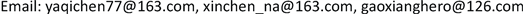Pure Mathematics
Vol. 09  No. 03 ( 2019 ), Article ID: 30406 , 6 pages
10.12677/PM.2019.93057

Some Hadamard-Type Inequalities for (h, m)-Convex Functions

Yaqi Chen, Xin Chen, Xiang Gao

School of Mathematics Sciences, Ocean University of China, Qingdao ShandongReceived: Apr. 26th, 2019; accepted: May 6th, 2019; published: May 24th, 2019ABSTRACT

In this paper, we are interested to present the Hermite-Hadamard-type inequalities for product of (h, m)-convex functions via three new inequalities. Presented results have close connection with some classical Hermite-Hadamard-type inequality.1. 引言

2. 定义

2.1. 凸函数

2.2. h-凸函数

2.3. m-凸函数

2.4. (h, m)-凸函数

$f\left(\frac{a+b}{2}\right)\le \frac{1}{b-a}{\int }_{a}^{b}f\left(x\right)\text{d}x\le \frac{f\left(a\right)+f\left(b\right)}{2}$ (1)

3. 引理

3.1. 引理1 

$\frac{\text{1}}{b-a}{\int }_{a}^{b}f\left(x\right)g\left(x\right)\text{d}x\le \frac{1}{3}M\left(a,b\right)+\frac{1}{6}N\left(a,b\right)$ (2)

$2f\left(\frac{a+b}{2}\right)g\left(\frac{a+b}{2}\right)\le \frac{1}{b-a}{\int }_{a}^{b}f\left(x\right)g\left(x\right)\text{d}x+\frac{1}{6}M\left(a,b\right)+\frac{1}{3}N\left(a,b\right)$ (3)

3.2. 引理2 

$\frac{1}{b-a}{\int }_{a}^{b}f\left(x\right)g\left(x\right)\text{d}t\le M\left(a,b\right){\int }_{0}^{1}{h}_{1}\left(t\right){h}_{2}\left(t\right)\text{d}t+N\left(a,b\right){\int }_{0}^{1}{h}_{1}\left(t\right){h}_{2}\left(1-t\right)\text{d}t$ (4)

3.3. 引理3 

$f\left(\frac{a+b}{2}\right)g\left(\frac{a+b}{2}\right)-\frac{2}{b-a}{h}_{1}\left(\frac{1}{2}\right){h}_{2}\left(\frac{1}{2}\right)\le {h}_{1}\left(\frac{1}{2}\right){h}_{2}\left(\frac{1}{2}\right)\left[N\left(a,b\right){\int }_{0}^{1}{h}_{1}\left(t\right){h}_{2}\left(t\right)\text{d}t+M\left(a,b\right){\int }_{0}^{1}{h}_{1}\left(t\right){h}_{2}\left(1-t\right)\text{d}t\right]$ (5)

3.4. 引理4 

$\begin{array}{c}\frac{\text{1}}{\text{2}h\left(\frac{1}{2}\right)}f\left(\frac{a+b}{2}\right)\le \frac{1}{b-a}{\int }_{a}^{b}f\left(x\right)\text{d}x\\ \le \left[f\left(a\right)+f\left(b\right)\right]{\int }_{0}^{1}h\left(t\right)\text{d}t\end{array}$ (6)

4. 主要结果

4.1. 定理1

$f,g$ 是定义在区间I上的函数，其中f是 $\left({h}_{1},m\right)$ -凸函数，g是 $\left({h}_{2},m\right)$ -凸函数，且 $fg\in {L}_{1}\left(\left[a,b\right]\right)$${h}_{1}{h}_{2}\in {L}_{1}\left(\left[0,1\right]\right)$$a,b\in I$，其中 $a，且 $f\in {L}_{1}\left(\left[a,b\right]\right),t\in \left[0,1\right]$，则有以下结果成立：

$\frac{\text{1}}{mb-a}{\int }_{a}^{mb}f\left(x\right)g\left(x\right)\text{d}t\le M\left(a,b\right){\int }_{0}^{1}{h}_{1}\left(t\right){h}_{2}\left(t\right)\text{d}t+N\left(a,b\right){\int }_{0}^{1}{h}_{1}\left(t\right){h}_{2}\left(1-t\right)\text{d}t$

$f\left(ta+m\left(1-t\right)b\right)\le {h}_{1}\left(t\right)f\left(a\right)+m{h}_{1}\left(1-t\right)f\left(b\right)$ (7)

$g\left(ta+m\left(1-t\right)b\right)\le {h}_{2}\left(t\right)g\left(a\right)+m{h}_{2}\left(1-t\right)g\left(b\right)$ (8)

$\begin{array}{l}f\left(ta+m\left(1-t\right)b\right)g\left(ta+m\left(1-t\right)b\right)\\ \le \left({h}_{1}\left(t\right)f\left(a\right)+m{h}_{1}\left(1-t\right)f\left(b\right)\right)×\left({h}_{2}\left(t\right)g\left(a\right)+m{h}_{2}\left(1-t\right)g\left(b\right)\right)\end{array}$

$\begin{array}{l}f\left(ta+m\left(1-t\right)b\right)g\left(ta+m\left(1-t\right)b\right)\\ \le {h}_{1}\left(t\right){h}_{2}\left(t\right)f\left(a\right)g\left(a\right)+\left[m{h}_{1}\left(t\right){h}_{2}\left(1-t\right)f\left(a\right)g\left(b\right)\\ \text{\hspace{0.17em}}\text{\hspace{0.17em}}\text{ }×m{h}_{1}\left(1-t\right){h}_{2}\left(t\right)f\left(a\right)g\left(a\right)\right]+{m}^{2}{h}_{1}\left(1-t\right){h}_{2}\left(1-t\right)f\left(b\right)g\left(b\right)\end{array}$

$\begin{array}{l}{\int }_{0}^{1}f\left(ta+m\left(1-t\right)b\right)g\left(ta+m\left(1-t\right)b\right)\text{d}t\\ \le \left[f\left(a\right)g\left(a\right)+{m}^{2}f\left(b\right)g\left(b\right)\right]{\int }_{0}^{1}{h}_{1}\left(t\right){h}_{2}\left(t\right)\text{d}t\\ \text{\hspace{0.17em}}\text{\hspace{0.17em}}\text{ }\text{ }+m\left[f\left(a\right)g\left(b\right)+f\left(b\right)g\left(a\right)\right]{\int }_{0}^{1}{h}_{1}\left(t\right){h}_{2}\left(1-t\right)\text{d}t\end{array}$

$x=ta+m\left(1-t\right)b$，整理后得到：

$\begin{array}{l}\frac{\text{1}}{mb-a}{\int }_{a}^{m}bf\left(x\right)g\left(x\right)\text{d}t\\ \le M\left(a,b\right){\int }_{0}^{1}{h}_{1}\left(t\right){h}_{2}\left(t\right)\text{d}t+N\left(a,b\right){\int }_{0}^{\text{1}}{h}_{1}\left(t\right){h}_{2}\left(1-t\right)\text{d}t\end{array}$

4.2. 定理2

$f,g$ 是定义在区间I上的函数，其中f是 $\left({h}_{1},m\right)$ -凸函数，g是 $\left({h}_{2},m\right)$ -凸函数，且 $fg\in {L}_{1}\left(\left[a,b\right]\right)$ ${h}_{1}{h}_{2}\in {L}_{1}\left(\left[0,1\right]\right)$$a,b\in I$，其中 $a，且 $f\in {L}_{1}\left(\left[a,b\right]\right),t\in \left[0,1\right]$，则有以下结果成立：

$\begin{array}{l}f\left(\frac{a+mb}{2}\right)g\left(\frac{a+mb}{2}\right)-\frac{1+{m}^{2}}{mb-a}{h}_{1}\left(\frac{1}{2}\right){h}_{2}\left(\frac{1}{2}\right){\int }_{a}^{mb}f\left(x\right)g\left(x\right)\text{d}x\\ \le 2m{h}_{1}\left(\frac{1}{2}\right){h}_{2}\left(\frac{1}{2}\right)\left[N\left(a,b\right){\int }_{0}^{1}{h}_{1}\left(t\right){h}_{2}\left(t\right)\text{d}t+M\left(a,b\right){\int }_{0}^{1}{h}_{1}\left(t\right){h}_{2}\left(1-t\right)\text{d}t\right]\end{array}$

$\begin{array}{l}{\int }_{0}^{1}f\left(at+m\left(1-t\right)b\right)g\left(at+m\left(1-t\right)b\right)\text{d}t+{\int }_{0}^{1}{m}^{2}f\left(\left(1-t\right)a+mtb\right)g\left(\left(1-t\right)a+mtb\right)\text{d}t\\ =\frac{1+{m}^{2}}{mb-a}{\int }_{a}^{mb}f\left(x\right)g\left(x\right)\text{d}x\end{array}$

$\begin{array}{l}f\left(\frac{a+mb}{2}\right)g\left(\frac{a+mb}{2}\right)-\frac{1+{m}^{2}}{mb-a}2{h}_{1}\left(\frac{1}{2}\right){h}_{2}\left(\frac{1}{2}\right){\int }_{a}^{mb}f\left(x\right)g\left(x\right)\text{d}x\\ \le 2m{h}_{1}\left(\frac{1}{2}\right){h}_{2}\left(\frac{1}{2}\right)×\left[M\left(a,b\right){\int }_{0}^{1}{h}_{1}\left(t\right){h}_{2}\left(t\right)\text{d}t+M\left(a,b\right){\int }_{0}^{1}{h}_{1}\left(t\right){h}_{2}\left(1-t\right)\text{d}t\right]\end{array}$

4.3. 定理3

$\frac{1}{\left(\text{1}+m\right)h\left(\frac{1}{2}\right)}f\left(\frac{a+mb}{2}\right)\le \frac{1}{mb-a}{\int }_{a}^{mb}f\left(x\right)\text{d}x\le \left[f\left(a\right)+mf\left(b\right)\right]{\int }_{0}^{1}h\left(t\right)\text{d}t$

$\frac{1}{mb-a}{\int }_{a}^{mb}f\left(x\right)\text{d}x\le {\int }_{0}^{1}h\left(t\right)f\left(a\right)\text{d}t+{\int }_{0}^{1}mh\left(t\right)f\left(b\right)\text{d}t=\left[f\left(a\right)+mf\left(b\right)\right]{\int }_{0}^{1}h\left(t\right)\text{d}t$

$x=ta+m\left(1-t\right)b,y=\left(1-t\right)a+mtb$，可以得到，

$f\left(\frac{a+mb}{2}\right)=f\left(\frac{x+y}{2}\right)\le h\left(\frac{1}{2}\right)\left[f\left(ta+m\left(1-t\right)b\right)+mf\left(\left(1-t\right)a+mtb\right)\right]$

$\begin{array}{c}f\left(\frac{a+mb}{2}\right)\le h\left(\frac{1}{2}\right)\frac{1}{mb-a}{\int }_{a}^{mb}f\left(x\right)\text{d}x+h\left(\frac{1}{2}\right)\frac{m}{mb-a}{\int }_{a}^{mb}f\left(x\right)\text{d}x\\ =h\left(\frac{1}{2}\right)\frac{1+m}{mb-a}{\int }_{a}^{mb}f\left(x\right)\text{d}x\end{array}$

$\frac{1}{\left(1+m\right)h\left(\frac{1}{2}\right)}f\left(\frac{a+mb}{2}\right)\le \frac{1}{mb-a}{\int }_{a}^{mb}f\left(x\right)\text{d}x\le \left[f\left(a\right)+mf\left(b\right)\right]{\int }_{0}^{1}h\left(t\right)\text{d}t$

Some Hadamard-Type Inequalities for (h, m)-Convex Functions[J]. 理论数学, 2019, 09(03): 421-426. https://doi.org/10.12677/PM.2019.93057

1. 1. Hadamard, J. (1893) Etude sur les proprits des fonctions entires et en particulier d’une fonction considre par Riemann. Journal de Mathmatiques Pures et Appliques, 58, 171-215.

2. 2. Pachpatte, B.G. (2003) On Some Inequalities for Convex Functions. Journal of Mathematical Inequalities, 3, 315-321.

3. 3. Sarikaya, M.Z., Saglam, A. and Yildirim, H. (2008) On Some Hadamard-Type Inequalities for h-Convex Functions. Journal of Mathematical Inequalities, 2, 335-341.
https://doi.org/10.7153/jmi-02-30